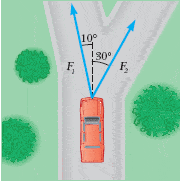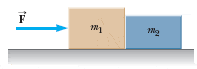First, I'd like to say I'm new here, but have browsed the forums since the beginning of this semester for previously worked problems. I'm finding my textbook somewhat useless in helping me to understand how to solve some of the hw problems. My first semester in Physics so please be nice to naivete.

## Homework Statement

Two forces are applied to a car in an effort to move it, as shown in the following figure, where F1 = 401 N and F2 = 378 N. (Assume up and to the right as positive directions.)a) What is the resultant of the two forces? Give magnitude (N) and direction (in degrees to the right of the forward direction)

b) If the car has a mass of 3,000 kg, what acceleration does it have? Ignore friction.

## Homework Equations

I'm not given any since there aren't any directly relative problems in my text, but I tried using some information on formulas I got during lecture after making a force body diagram. I'm not sure how to find direction, and the answers I got thus far were reported to be incorrect.

X direction:
F1x=401sin10*
F2x=378sin30*
F2x-F1x=ma

Y direction:
F1y=401cos10*
F2y=378cos30*
F2y+F1y=ma

Constant a=(F2x-F1x/3000)+(F2y+F1y/3000)

## The Attempt at a Solution

rounded...

F1x=189
F2x=70
so for a in x direction, I got: (69.6-189)/3000 = -.0397 m/s2

F1y=395
F2y=327
for a in y direction, I got (395+327)/3000 = .2407

and for constant a = .201

That is what I have so far for my first problem question. For my second problem question, it's throwing me off partly because there are no #'s at all. I'm totally lost on it.

#2) Homework Statement

Two blocks of masses m1 and m2 (m1 > m2) are placed on a frictionless table in contact with each other. A horizontal force of magnitude F is applied to the block of mass m1 in the figure below. P is the magnitude of the contact force between the blocks.a) What is the net force on the system consisting of both blocks? Use P and F as necessary.

b)What is the net force acting on M1? Use P and F

c) What is the net force on M2? Use P and F.

d) Find acceleration a and contact force P, in terms of F, m1, and m2.

I'm honestly not at all sure what to do for this one. There's literally no example of one object pushing on another in my book, and we didn't go over it in lecture. Any help you can provide me with on any of these problems would be greatly appreciated. I'm sorry if this seem incredibly easy to any of you, but I'm just starting out. I was able to answer the other 7 hw questions so far, and haven't had any trouble with the previous chapters (1D motion, 2D motion & vectors, and projectile motion).

berkeman
Mentor

First, I'd like to say I'm new here, but have browsed the forums since the beginning of this semester for previously worked problems. I'm finding my textbook somewhat useless in helping me to understand how to solve some of the hw problems. My first semester in Physics so please be nice to naivete.

## Homework Statement

Two forces are applied to a car in an effort to move it, as shown in the following figure, where F1 = 401 N and F2 = 378 N. (Assume up and to the right as positive directions.)a) What is the resultant of the two forces? Give magnitude (N) and direction (in degrees to the right of the forward direction)

b) If the car has a mass of 3,000 kg, what acceleration does it have? Ignore friction.

## Homework Equations

I'm not given any since there aren't any directly relative problems in my text, but I tried using some information on formulas I got during lecture after making a force body diagram. I'm not sure how to find direction, and the answers I got thus far were reported to be incorrect.

X direction:
F1x=401sin10*
F2x=378sin30*
F2x-F1x=ma

Y direction:
F1y=401cos10*
F2y=378cos30*
F2y+F1y=ma

Constant a=(F2x-F1x/3000)+(F2y+F1y/3000)

## The Attempt at a Solution

rounded...

F1x=189
F2x=70
so for a in x direction, I got: (69.6-189)/3000 = -.0397 m/s2

F1y=395
F2y=327
for a in y direction, I got (395+327)/3000 = .2407

and for constant a = .201

That is what I have so far for my first problem question. For my second problem question, it's throwing me off partly because there are no #'s at all. I'm totally lost on it.

#2) Homework Statement

Two blocks of masses m1 and m2 (m1 > m2) are placed on a frictionless table in contact with each other. A horizontal force of magnitude F is applied to the block of mass m1 in the figure below. P is the magnitude of the contact force between the blocks.a) What is the net force on the system consisting of both blocks? Use P and F as necessary.

b)What is the net force acting on M1? Use P and F

c) What is the net force on M2? Use P and F.

d) Find acceleration a and contact force P, in terms of F, m1, and m2.

I'm honestly not at all sure what to do for this one. There's literally no example of one object pushing on another in my book, and we didn't go over it in lecture. Any help you can provide me with on any of these problems would be greatly appreciated. I'm sorry if this seem incredibly easy to any of you, but I'm just starting out. I was able to answer the other 7 hw questions so far, and haven't had any trouble with the previous chapters (1D motion, 2D motion & vectors, and projectile motion).

Pretty good work on the first problem. But keep in mind that the car will only roll in the y direction, so there is no acceleration in the x direction.

On the 2nd problem, draw free-body diagrams (FBDs) for each object (the two boxes), and label all the forces that act on each...

Thank you so much for your response. For question 1, how can I determine the direction in degrees?

And since x direction doesnt really matter, my acceleration came out to .2407 m/s2 which was indicated to be correct - thank you for your help on that!

For problem 2, I made an f body diagram but I'm still not entirely sure how it all ties together...

would net force = (m1+m2)(2P+F) ? am I even close or way off the mark? I believe once I understand one part of this system, that I can figure out all of the other parts. All I've got for finding the acceleration in this problem is: a=(m1+m2)/F

berkeman
Mentor

Thank you so much for your response. For question 1, how can I determine the direction in degrees?

You convert from the rectangular form of the resultant vector to polar form, except that they say to give the degrees to the right of up in the drawing. Are you familiar with how to convert back and forth between rectangular and polar form for vectors?

For problem 2, I made an f body diagram but I'm still not entirely sure how it all ties together...

would net force = (m1+m2)(2P+F) ? am I even close or way off the mark? I believe once I understand one part of this system, that I can figure out all of the other parts. All I've got for finding the acceleration in this problem is: a=(m1+m2)/F

Always keep using F = ma ... I'm not sure what "net force = (m1+m2)(2P+F)" means.

But your last part is right. The net force pushes both masses, so the acceleration is based on both. And when it asks for the force between the blocks, the left block is pusing the right block, so what would you use for the mass in that F=ma calculation?

Thanks again for the prompt and helpful response. And thank you for your patience with my very limited physical knowledge

I'm not familiar with converting interchangably from rectangular to polar vectors. Is there a basic formula?

In respect to problem 2, I'm having trouble trying to come up with a way to define net force without using 'a' since it only tells me to use p and f. So that first jumble was my attempt at it.

For your second question, I'm guessing for that part I'd say F=m1P*m2*a ?

berkeman
Mentor

I'm not familiar with converting interchangably from rectangular to polar vectors. Is there a basic formula?

This wikipedia link should help: http://en.wikipedia.org/wiki/Polar_coordinate_system

In respect to problem 2, I'm having trouble trying to come up with a way to define net force without using 'a' since it only tells me to use p and f. So that first jumble was my attempt at it.

For your second question, I'm guessing for that part I'd say F=m1P*m2*a ?

I'd do 3 FBDs. One for each mass, and one for the combined masses. The masses do not come apart, so they both have the same acceleration (all 3 FBDs). But since they have different masses, that means from F=ma that they each experience different net force.

What is the net force on the combination of masses in terms of the acceleration a?

So what is the net force on the mass m2 in terms of a?

And what is the net force on the mass m1 in terms of a? Why is it less than F?